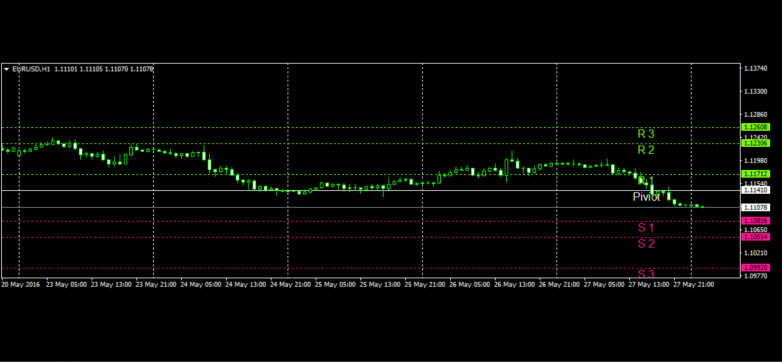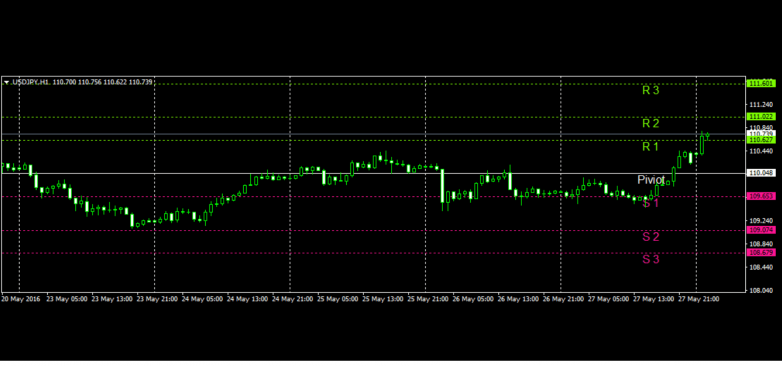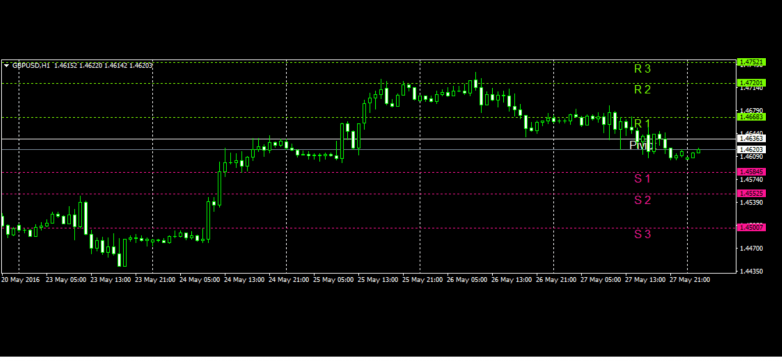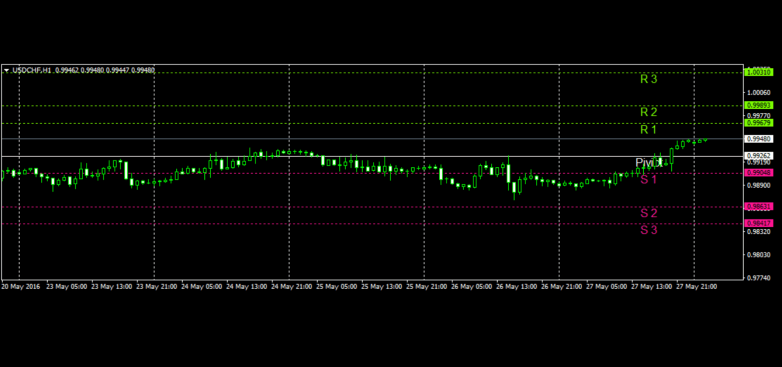HiWayFX - Analytics

## HiWayFX

0.00 9.25/10
33% of positive reviews

# Technical Analysis - 30/05/2016

## EURUSD

The EURUSD pair closed on Friday, 27th of May 2016, at 1.11137. A breakout below daily pivot which is 1.11410 will technically lead us to S1 = 1.10816, and later to S2 = 1.10514. Overextension of price will lead us to S3, which is 1.09920. A breakout above daily pivot which is 1.11410 will technically lead us to R1 = 1.11712 and later to R2 = 1.12306. Overextension of price will lead us to R3, which is 1.12608. Exponential moving average of 21 periods indicates a bullish price movement.

### Pivot Point 1 Hour

 S3 S2 S1 Daily Pivot R1 R2 R3 Classic 1.09920 1.10514 1.10816 1.11410 1.11712 1.12306 1.12608## USDJPY

The USDJPY pair closed on Friday, 27th of May 2016, at 110.408. A breakout above daily pivot which is 110.048 will technically lead us to R1 = 110.627 and later to R2 = 111.022 Overextension of price will lead us to R3, which is 111.601. A breakout below daily pivot which is 110.048 will technically lead us to S1 = 109.653, and later to S2 = 109.074. Overextension of price will lead us to S3, which is 108.679. Exponential moving average of 21 periods indicates a bearish price movement.

### Pivot Point 1 Hour

 S3 S2 S1 Daily Pivot R1 R2 R3 Classic 108.679 109.074 109.653 110.048 110.627 111.022 111.601## GBPUSD

The GBPUSD pair closed Friday, 27th of May 2016, at 1.46085. A breakout below daily pivot which is 1.46363 will technically lead us to S1 = 1.45845 and later to S2 = 1.45525. Overextension of price will lead us to S3, which is 1.45007. A breakout above daily pivot which is 1.46363 will technically lead us to R1 = 1.46683, and later to R2 = 1.47201. Overextension of price will lead us to R3, which is 1.47521. Exponential moving average of 21 periods indicates a bearish price movement.

### Pivot Point 1 Hour

 S3 S2 S1 Daily Pivot R1 R2 R3 Classic 1.45007 1.45525 1.45845 1.46363 1.46683 1.47201 1.47521## USDCHF

The USDCHF pair closed on Friday, 27th of May 2016, at 0.99448 A breakout above daily pivot which is 0.99262 will technically lead us to R1 = 0.99679 and later to R2 = 0.99893. Overextension of price will lead us to R3, which is 0.00310. A breakout below daily pivot which is 0.99262 will technically lead us to S1 = 0.99048, and later to S2 = 0.98631. Overextension of price will lead us to S3, which is 0.98417. Exponential moving average of 21 periods indicates a bearish price movement.

### Pivot Point 1 Hour

 S3 S2 S1 Daily Pivot R1 R2 R3 Classic 0.98417 0.98631 0.99048 0.99262 0.99679 0.99893 0.00310